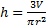# Solving Formulas for Different Variables

 Often it is useful to solve a formula for a different variable, for example, the formula for speed is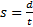, but if you are looking for the distance (d), it would be helpful to have the formula solved for d as in: d=st. Remember that to solve for a variable means that you have to get that variable by itself. To solve a formula for a different variable, you use the same process as you would for a regular equation. If a variable is added, you add the opposite to both sides. If it is multiplied or divided, you do the inverse operation. The only difference is that you are using variables instead of numbers. For example, let's solve I=Prt (the interest formula) for t. We need to move the P and the r in order to get t by itself. We could move both in one step, but we'll do them one at a time to be extra clear. Let's move the P first. The P is being multiplied by the t, so we have to do the reverse: divide both sides by P.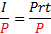The P's on the right hand side will cancel each other out and we are left with: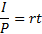We still have to move the r. It is also being multiplied, so we will divide both side by r.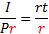The r's on the right will cancel, and we have t by itself. We can reverse the sides of the equation if we want.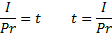And that's the answer. We now have the equation solved for t instead of I. Let's try one more: Let's solve the formula for the area of a triangle f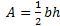for b. We need to move the h and thein order to get b by itself. Let's move the h first. The h is being multiplied by the b, so we have to do the reverse: divide both sides by h.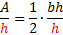The h's on the right hand side will cancel each other out and we are left with: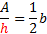We still have to move the. It is also being multiplied, so we will divide both side by. Remember that to divide by a fraction, you flip it and multiply, so we will flip, which gives us 2. We will now multiply both sides by 2.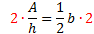The 2's on the right will cancel, and we have b by itself. We can reverse the sides of the equation if we want.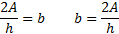Practice:Solve each formula for the given variable. 1) Solve I=Prt for P. 2) Solve A=bh for b. 3) Solve C=2Πr for r. 4) Solve F=ma for m. 5) Solve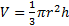for h. Answers: 1)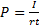2)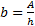3)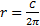4)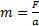5)